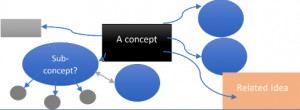# Concept Maps: a useful way of putting theory into practice

In an engineering degree, students start by learning fundamental mathematical principles. In second year, students are expected to make a substantial conceptual leap from “what maths is” to “how to use maths in engineering”. Putting first principles into action can be particularly challenging for students, especially with subjects such as fluid mechanics, solid mechanics, and dynamics.

In Fluid Mechanics I (AMME2261) a set of partial differential equations, known as the Navier-Stokes (N-S) equations, are introduced early in second year. Despite a large number of worked examples completed on this topic every year, it is clear that many students still find it very challenging to accurately apply the N-S equations to practical scenarios.

## Would seeing connections be more helpful for the students?In AMME2261 taught by Dr Agi Kourmatzis, an instructor-created concept map for the N-S equations was introduced in week 7. Students were subsequently encouraged to create their own concept maps for the same topic and/or earlier topics.

Concept maps are a way to graphically represent ideas and how they relate to each other. They have been shown to be better for students’ ability to retain complex theories than simply reading or attending lectures, or creating summaries or outlines. Concept maps allow students to create their own meaning of the learning content and demonstrate their understanding of the connections between ideas. This can be particularly useful when students are presented with novel topics in higher education.

The topic chosen for the introduction of concept maps was fundamental fluid dynamics, which takes place from weeks 5 – 7. An instructor-created concept map was presented to students to consolidate knowledge related to the N-S equations.

The instructor-created concept map itself was comprised of a visual sequence of interconnected steps (“nodes”) for approaching an N-S problem. Each “node” in the concept map represents different assumptions, situations, or concepts which were learnt in previous weeks. Solving an N-S equation always involves starting from a complex set of partial differential equations (the N-S equations) and then making certain assumptions to remove terms from the equations, therefore simplifying them considerably.

If the student follows the concept map correctly then a final simplified form of the N-S equations will result.

In previous semesters, instead of a visual map, multiple worked examples were done using the N-S equations with an instructor-generated list provided during a revision lecture. This approach is still the main method of teaching this part of the unit in the tutorials, but the concept mapping approach has now been adopted within lectorial sessions which run every week. Retaining both methods of teaching the N-S equations, i.e. worked examples in tutorials & concept mapping in lectorials, is essential given that it is widely understood that students learn in different ways.

## Results and next steps

### Quiz and exam performance

Quiz 3 this year was run similarly to previous years, with a long answer question which assessed use of the N-S equations in fluid dynamics. The average grades for the quiz were calculated and compared to previous years. Grades from 2018 onwards were used, enabling comparison between 5 cohorts. In 2022, there was a marked increase in overall grades when compared to 2019, 2020 and 2021, with the highest percentage of distinctions noted compared to all previous years.

A similar analysis was also performed on the N-S exam question from the 2020 exam onwards (which is when the exam became open book and fully online). Again, results indicate there was a significant increase in grades in 2022.

Overall, there is significant and substantial evidence from both the quiz and the final exam that students generally performed better on the N-S equation problem this year. This strongly indicates that students benefitted from visualising interconnections related to the N-S equation, allowing them to make connections between the stages.

### Student responses

Students found the instructor-generated concept map useful, but they did not engage with creating their own concept maps. Informal feedback from students indicated they did not feel comfortable developing their own concept maps, and were concerned they would ‘get it wrong’.

In semester 1, 2023 we are introducing concept maps earlier in the semester and embedding it as a formative assessment, to better scaffold its integration into the unit of study. We will also be including student-created concept mapping activities in order to encourage constructivist and active learning.

From the above, we can see that concept maps are a useful way of presenting complex material and a useful way for students to consolidate knowledge.

## Tell me more!

For more information or to discuss how concept maps might work in your units of study, please feel free to get in touch with Peter Lok, Educational Designers in the Faculty of Engineering. To discuss Fluid Dynamics and the Navier-Stokes Equation, please feel free to get in touch with Agi Kourmatzis

This is an extract from a conference paper “A proposed methodology and reflection for the use of concept maps in fundamental fluid dynamics” presented at AAEE2022 Future of Engineering Education in December 2022, at Western Sydney University.